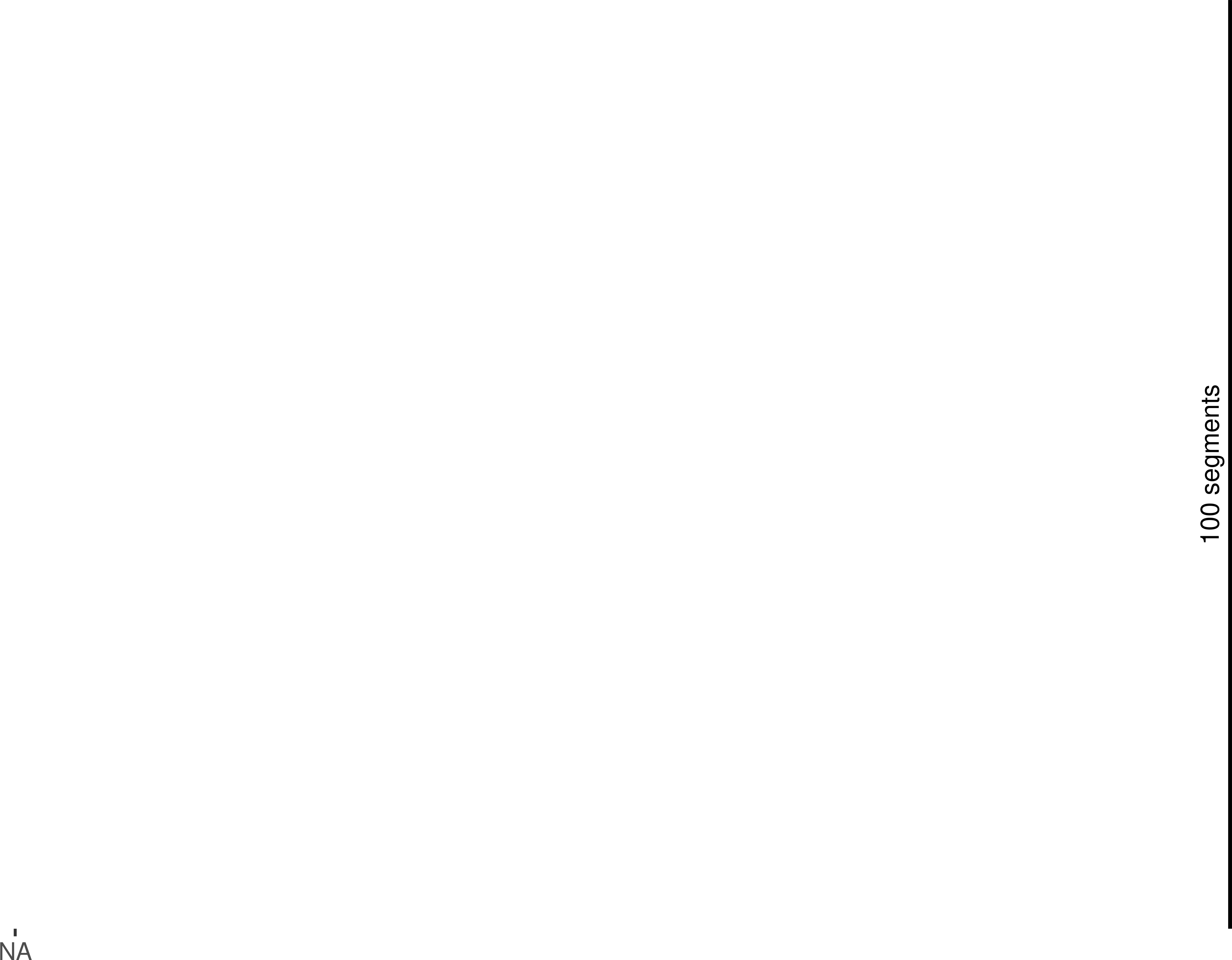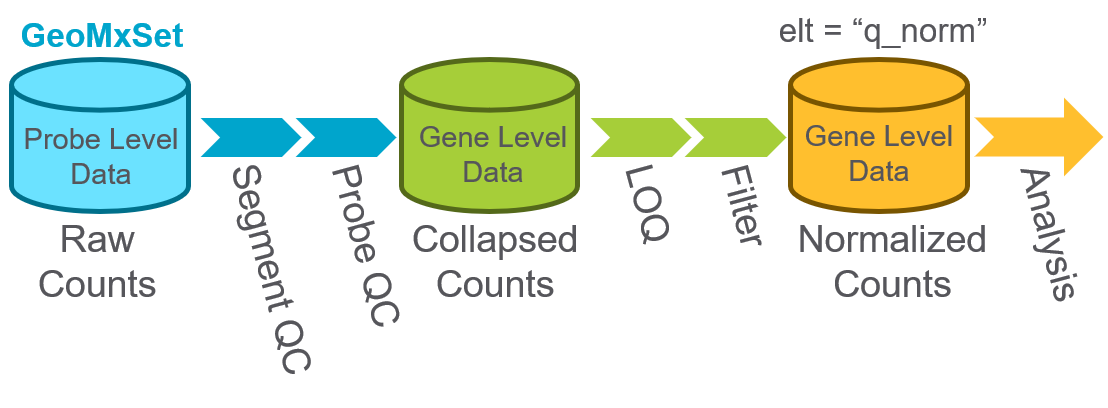R version: R version 4.3.0 RC (2023-04-13 r84269)
Bioconductor version: 3.17
Package: 3.4.0 <>

# 1 Introduction

In this vignette, we will introduce a data analysis workflow for GeoMx-NGS mRNA expression data.

The GeoMx Digital Spatial Profiler (DSP) is a platform for capturing spatially resolved high-plex gene (or protein) expression data from tissue Merritt et al., 2020. In particular, formalin-fixed paraffin-embedded (FFPE) or fresh-frozen (FF) tissue sections are stained with barcoded in-situ hybridization probes that bind to endogenous mRNA transcripts. The user then selects regions of the interest (ROI) to profile; if desired, each ROI segment can be further sub-divided into areas of illumination (AOI) based on tissue morphology. The GeoMx then photo-cleaves and collects expression barcodes for each AOI segment separately for downstream sequencing and data processing.

The final results are spatially resolved unique expression datasets for every protein-coding gene (>18,000 genes) from every individual segment profiled from tissue.

## 1.1 Motivation & Scope

The motivation for this vignette is to enable scientists to work with GeoMx-NGS gene expression data and understand a standard data analysis workflow.

Our specific objectives:

• Load GeoMx raw count files and metadata (DCC, PKC, and annotation file)
• Perform quality control (QC), filtering, and normalization to prepare the data
• Perform downstream visualizations and statistical analyses including:
• Dimension reduction with UMAP or t-SNE
• Heatmaps and other visualizations of gene expression
• Differential expression analyses with linear mixed effect models

# 2 Getting started

Let’s install and load the GeoMx packages we need:

``````if (!require("BiocManager", quietly = TRUE))
install.packages("BiocManager")

# The following initializes most up to date version of Bioc
BiocManager::install(version="3.15")

BiocManager::install("NanoStringNCTools")
BiocManager::install("GeomxTools")
BiocManager::install("GeoMxWorkflows")``````
``````library(NanoStringNCTools)
library(GeomxTools)
library(GeoMxWorkflows)``````
``````if(packageVersion("GeomxTools") < "2.1" &
packageVersion("GeoMxWorkflows") >= "1.0.1"){
stop("GeomxTools and Workflow versions do not match. Please use the same version.
This workflow is meant to be used with most current version of packages.
If you are using an older version of Bioconductor please reinstall GeoMxWorkflows and use vignette(GeoMxWorkflows) instead")
}

if(packageVersion("GeomxTools") > "2.1" &
packageVersion("GeoMxWorkflows") <= "1.0.1"){
stop("GeomxTools and Workflow versions do not match.
Please use the same version, see install instructions above.")

# to remove current package version
# remove.packages("GeomxTools")
# remove.packages("GeoMxWorkflows")
# see install instructions above
}``````

In this vignette, we will analyze a GeoMx kidney dataset created with the human whole transcriptome atlas (WTA) assay. The dataset includes 4 diabetic kidney disease (DKD) and 3 healthy kidney tissue samples. Regions of interest (ROI) were spatially profiled to focus on two different kidney structures: tubules or glomeruli. One glomerular ROI contains the entirety of a single glomerulus. Each tubular ROI contains multiple tubules that were segmented into distal (PanCK+) and proximal (PanCK-) tubule areas of illumination (AOI).

Download and the unzip the kidney data set found on the NanoString Website

The key data files are:

• DCCs files - expression count data and sequencing quality metadata
• PKCs file(s) - probe assay metadata describing the gene targets present in the data, PKC files can be found here
• Annotation file - useful tissue information, including the type of segment profiled (ex: glomerulus vs. tubule), segment area/nuclei count, and other tissue characteristics (ex: diseased vs. healthy). If working with a new dataset, use the lab worksheet from the GeoMx instrument study readout package, as the annotation order of NTCs is important to ensure proper processing of files.

``````# Reference the main folder 'file.path' containing the sub-folders with each
# data file type:
package="GeoMxWorkflows")
# to locate a specific file path replace the above line with
# replace the Folder, SubFolder, DataLocation as needed

# the DataLocation folder should contain a dccs, pkcs, and annotation folder
# with each set of files present as needed``````
``````# automatically list files in each directory for use
DCCFiles <- dir(file.path(datadir, "dccs"), pattern = ".dcc\$",
full.names = TRUE, recursive = TRUE)
PKCFiles <- unzip(zipfile = dir(file.path(datadir, "pkcs"), pattern = ".zip\$",
full.names = TRUE, recursive = TRUE))
SampleAnnotationFile <-
full.names = TRUE, recursive = TRUE)``````

We then load the data to create a data object using the `readNanoStringGeoMxSet` function.

``````# load data
demoData <-
pkcFiles = PKCFiles,
phenoDataFile = SampleAnnotationFile,
phenoDataSheet = "Template",
protocolDataColNames = c("aoi", "roi"),
experimentDataColNames = c("panel"))``````

All of the expression, annotation, and probe information are now linked and stored together into a single data object.

For more details on this object’s structure and accessors, please refer to the “GeoMxSet Object Overview” section at the end of this vignette.

# 3 Study Design

## 3.1 Modules Used

First let’s access the PKC files, to ensure that the expected PKCs have been loaded for this study. For the demo data we are using the file `Hsa_WTA_1.0.pkc`.

``````library(knitr)
pkcs <- annotation(demoData)
modules <- gsub(".pkc", "", pkcs)
kable(data.frame(PKCs = pkcs, modules = modules))``````
PKCs modules
Hsa_WTA_v1.0.pkc Hsa_WTA_v1.0

## 3.2 Sample Overview

Now that we have loaded the data, we can visually summarize the experimental design for our dataset to look at the different types of samples and ROI/AOI segments that have been profiled. We present this information in a Sankey diagram.

``````library(dplyr)
library(ggforce)

# select the annotations we want to show, use `` to surround column names with
# spaces or special symbols
count_mat <- count(pData(demoData), `slide name`, class, region, segment)
# simplify the slide names
count_mat\$`slide name` <- gsub("disease", "d",
gsub("normal", "n", count_mat\$`slide name`))
# gather the data and plot in order: class, slide name, region, segment
test_gr <- gather_set_data(count_mat, 1:4)
test_gr\$x <- factor(test_gr\$x,
levels = c("class", "slide name", "region", "segment"))
# plot Sankey
ggplot(test_gr, aes(x, id = id, split = y, value = n)) +
geom_parallel_sets(aes(fill = region), alpha = 0.5, axis.width = 0.1) +
geom_parallel_sets_axes(axis.width = 0.2) +
geom_parallel_sets_labels(color = "white", size = 5) +
theme_classic(base_size = 17) +
theme(legend.position = "bottom",
axis.ticks.y = element_blank(),
axis.line = element_blank(),
axis.text.y = element_blank()) +
scale_y_continuous(expand = expansion(0)) +
scale_x_discrete(expand = expansion(0)) +
labs(x = "", y = "") +
annotate(geom = "segment", x = 4.25, xend = 4.25,
y = 20, yend = 120, lwd = 2) +
annotate(geom = "text", x = 4.19, y = 70, angle = 90, size = 5,
hjust = 0.5, label = "100 segments")``````# 4 QC & Pre-processingThe steps above encompass the standard pre-processing workflow for GeoMx data. In short, they represent the selection of ROI/AOI segments and genes based on quality control (QC) or limit of quantification (LOQ) metrics and data normalization.

Before we begin, we will shift any expression counts with a value of 0 to 1 to enable in downstream transformations.

``````# Shift counts to one
demoData <- shiftCountsOne(demoData, useDALogic = TRUE)``````

## 4.1 Segment QC

We first assess sequencing quality and adequate tissue sampling for every ROI/AOI segment.

Every ROI/AOI segment will be tested for:

• % Aligned,% Trimmed, or % Stitched sequencing reads: segments below ~80% for one or more of these QC parameters are removed.
• % Sequencing saturation ([1-deduplicated reads/aligned reads]%): segments below ~50% require additional sequencing to capture full sample diversity and are not typically analyzed until improved.
• Negative Count: this is the geometric mean of the several unique negative probes in the GeoMx panel that do not target mRNA and establish the background count level per segment; segments with low negative counts (1-10) are not necessarily removed but may be studied closer for low endogenous gene signal and/or insufficient tissue sampling.
• No Template Control (NTC) count: values >1,000 could indicate contamination for the segments associated with this NTC; however, in cases where the NTC count is between 1,000- 10,000, the segments may be used if the NTC data is uniformly low (e.g. 0-2 counts for all probes).
• Nuclei: >100 nuclei per segment is generally recommended; however, this cutoff is highly study/tissue dependent and may need to be reduced; what is most important is consistency in the nuclei distribution for segments within the study.
• Area: generally correlates with nuclei; a strict cutoff is not generally applied based on area.

### 4.1.1 Select Segment QC

First, we select the QC parameter cutoffs, against which our ROI/AOI segments will be tested and flagged appropriately. We have selected the appropriate study-specific parameters for this study. Note: the default QC values recommended above are advised when surveying a new dataset for the first time.

``````# Default QC cutoffs are commented in () adjacent to the respective parameters
# study-specific values were selected after visualizing the QC results in more
# detail below
QC_params <-
percentTrimmed = 80,    # Minimum % of reads trimmed (80%)
percentStitched = 80,   # Minimum % of reads stitched (80%)
percentAligned = 75,    # Minimum % of reads aligned (80%)
percentSaturation = 50, # Minimum sequencing saturation (50%)
minNegativeCount = 1,   # Minimum negative control counts (10)
maxNTCCount = 9000,     # Maximum counts observed in NTC well (1000)
minNuclei = 20,         # Minimum # of nuclei estimated (100)
minArea = 1000)         # Minimum segment area (5000)
demoData <-
setSegmentQCFlags(demoData,
qcCutoffs = QC_params)

# Collate QC Results
QCResults <- protocolData(demoData)[["QCFlags"]]
flag_columns <- colnames(QCResults)
QC_Summary <- data.frame(Pass = colSums(!QCResults[, flag_columns]),
Warning = colSums(QCResults[, flag_columns]))
QCResults\$QCStatus <- apply(QCResults, 1L, function(x) {
ifelse(sum(x) == 0L, "PASS", "WARNING")
})
QC_Summary["TOTAL FLAGS", ] <-
c(sum(QCResults[, "QCStatus"] == "PASS"),
sum(QCResults[, "QCStatus"] == "WARNING"))``````

### 4.1.2 Visualize Segment QC

Before excluding any low-performing ROI/AOI segments, we visualize the distributions of the data for the different QC parameters. Note that the “Select Segment QC” and “Visualize Segment QC” sections are performed in parallel to fully understand low-performing segments for a given study. Iteration may follow to select the study-specific QC cutoffs.

For QC visualization, we write a quick function to draw histograms of our data.

``````library(ggplot2)

col_by <- "segment"

# Graphical summaries of QC statistics plot function
QC_histogram <- function(assay_data = NULL,
annotation = NULL,
fill_by = NULL,
thr = NULL,
scale_trans = NULL) {
plt <- ggplot(assay_data,
aes_string(x = paste0("unlist(`", annotation, "`)"),
fill = fill_by)) +
geom_histogram(bins = 50) +
geom_vline(xintercept = thr, lty = "dashed", color = "black") +
theme_bw() + guides(fill = "none") +
facet_wrap(as.formula(paste("~", fill_by)), nrow = 4) +
labs(x = annotation, y = "Segments, #", title = annotation)
if(!is.null(scale_trans)) {
plt <- plt +
scale_x_continuous(trans = scale_trans)
}
plt
}``````

Now we explore each of the QC metrics for the segments.

``QC_histogram(sData(demoData), "Trimmed (%)", col_by, 80)``